Name:    MCAS Electrical Systems

Multiple Choice
Identify the choice that best completes the statement or answers the question.

1.

An ammeter measures the current in the circuit shown below.When the piece of wire labeled X is replaced by a second identical R, which of the following happens to the current? (MCAS 2008)
 a. It increases. c. It goes to zero. b. It decreases. d. It remains unchanged.

2.

The power of a toaster can be determined if which of the following values are known? (MCAS 2008)
 a. the dimensions of the toaster b. the resistance of the toaster’s insulation c. the voltage applied to the toaster and the toaster’s temperature d. the current through the circuit and the voltage applied to the toaster

3.

Which of the following would be an advantage of using a 42 V battery in a car instead of a 12 V battery? (MCAS 2009)
 a. It would allow carmakers to use fewer circuits. b. It would eliminate the use of generators and alternators. c. It would produce the same amount of power using less current. d. It would produce the same amount of power using more current.

4.

A simple circuit is shown in the diagram below. (MCAS 2009)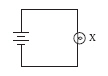In which of the following circuits will bulb X have the same brightness as in the circuit above, if all batteries and all light bulbs are identical?
 a.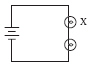b.c.d.5.

Students in a physics lab are studying the circuit shown in the diagram below. (MCAS 2004)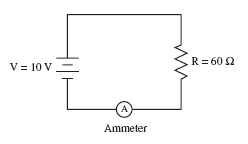Which of the following options will double the current through the ammeter?
 a. replacing the battery with a 5 V battery. c. replacing the resistor with a 30 W resistor. b. adding a 30 W resistor in parallel with R. d. adding a second 60 W resistor in series with R.

6.

A student is turning the handle of a generator. Another student connects a bulb to the generator. The bulb lights up. The first student continues to turn the handle of the generator at the same speed, but finds it harder to turn. Which of the following best explains this? (MCAS 2011)
 a. Less mechanical energy is required when the bulb is lit. b. More energy is put into the generator when the bulb is lit. c. Less mechanical energy is required the longer the generator is turned and the bulb is lit. d. The energy requirement of the generator is the same at any speed whether or not the bulb is lit.

7.

The table below provides information about the daily use of some typical appliances. (MCAS 2012)Which appliance uses the most energy?
 a. dishwasher b. air conditioner c. laptop computer d. clock radio

8.

The circuit shown below has two resistors in series.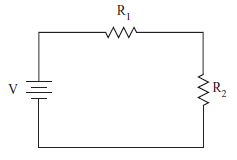Which of the following equations represents the current flowing through the two resistors? (MCAS 2014)
 a.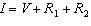b.c.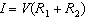d.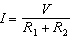9.

In the circuit shown below, a 12 V battery is connected in parallel to three resistors, labeled X, Y, and Z.Which of the following statements best describes the current flowing through the resistors in this circuit? (MCAS 2014)
 a. Resistor Y has the least current. b. Resistor X has the greatest current. c. Resistor Z has twice the current of resistor Y. d. Each resistor has the same amount of current flowing through it.

Problems

10.

A current of 2 A passes through two resistors placed in series. The first resistor has a resistance of 10 W and the second resistor has a resistance of 20 W . What is the total potential difference across the two resistors? (Modified MCAS 2007)

11.

The circuit diagram below shows three resistors, an ammeter, and a battery.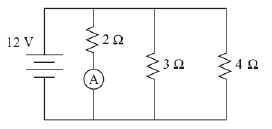How much current flows through the ammeter? (Modified MCAS 2006)

12.

A 72 W navigation unit on a commercial aircraft has a 24 V power supply and uses 3 A of electric current. What is the electrical resistance of the navigation unit? (Modified MCAS 2004)

13.

A circuit diagram is shown below.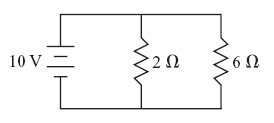What is the current through the 2W resistor? (Modified MCAS 2011)

14.

The potential difference across a resistor is 12 V. When a current of 5 A is flowing through this resistor, what is the power used by the resistor? (Modified MCAS 2011)

15.

A light bulb with a potential difference of 120 V across it carries a current of 1.5 A. Which of the following is the power consumption of the light bulb? (Modified MCAS 2013)

16.

The power supplied to a light bulb is 100 W. How much electrical energy is used by this light bulb in 20 s? (Modified MCAS 2014)

17.

The diagram below shows a circuit with a light bulb, an ammeter, and a 12 V battery.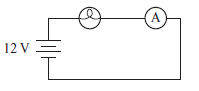The light bulb is lit for 30 s. The ammeter displays a current of 0.60 A.
What is the power rating for this circuit? (Modified MCAS 2014)

Open Response

18.

The diagram below shows a circuit with a 12 V battery connected in series with a 4 W resistor and a 2 W resistor. Two points in the circuit are labeled X and Y. (MCAS 2010)a. Calculate the current in the circuit. Show your calculations and include units in your answer.
b. Calculate the voltage drop across the 4 W resistor. Show your calculations and include units in your answer.
c. Determine the voltage drop between point X and point Y. Explain the reasoning for your answer.
d. Calculate the power of the entire circuit. Show your calculations and include units in your answer.

19.

The diagram below shows an incomplete parallel circuit and the components that will complete the circuit. (MCAS 2012)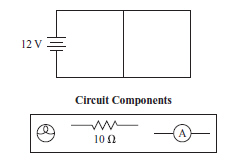a.       Re-draw the parallel circuit using all the circuit components shown. Place the ammeter in the circuit so that it measures the total current of the circuit.
b.       Calculate the current flowing through the resistor. Show your calculations and include units in your answer.

When the circuit is operating, the ammeter measures a total current of 1.5 A.

c.       Determine the resistance of the light bulb. Show your calculations or explain your reasoning. Include units in your answer.
d.       Describe one change that would result if the light bulb and resistor were placed in series instead of in parallel.

20.

A circuit diagram is shown below.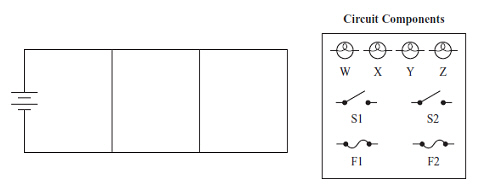Redraw this circuit diagram and add four identical light bulbs (W, X, Y, and Z), two switches (S1 and S2), and two fuses (F1 and F2). Your arrangement of these components must meet the requirements listed below. Be sure to read all of the requirements before adding the electrical components.
•       Light bulbs W and X have the same brightness.
•       Light bulbs Y and Z have the same brightness and are brighter than light bulbs W and X when all switches are closed.
•       The first switch (S1) controls only light bulbs W and X, and the second switch (S2) controls only light bulbs Y and Z.
•       The first fuse (F1) protects only light bulb Z, and the second fuse (F2) protects the entire circuit.

Use the circuit components shown above. Be sure to label all of the components with the labels given. (MCAS 2014)